# How to measure the termination angles on a Vogel cut crystal

The following is one method to measure the crystal's termination angles.

The tool needed for this job is an inexpensive (\$15) Digital Angle Finder Protractor. Ref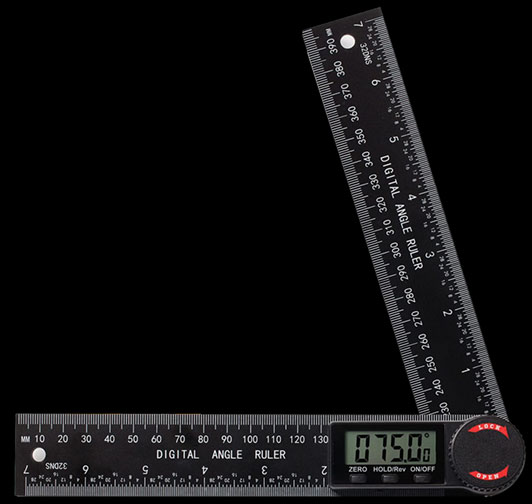Digital Angle Finder Protractor

60.0° emitter sending termination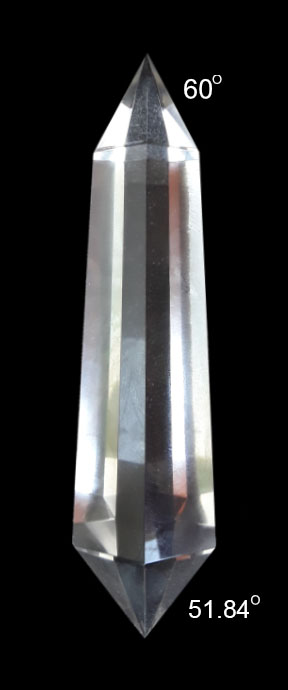51.84° transponding receiving termination

Let's say you want to determine how close the termination angles of 60° and 51.84° (commonly referred to as roughly 52°) and be able to measure how many degrees difference off they are from those ideal termination angles.

Note these angles were given by Jen Han Eredyon for cutting a 6 sided crystal in alignment with the hexagonal core of the lattice structure.

If your crystal is far off from these angles it does not mean your Vogel crystal is not effective. This is for reference only.

It is known that the three interior angles of a triangle will always have a sum of 180°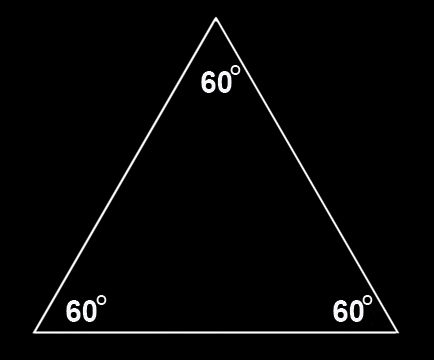60° + 60° + 60° = 180°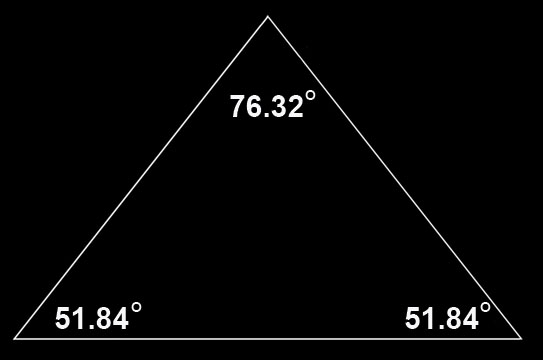51.84° + 51.84° + 76.32° = 180°

Therefore knowing that the angle of the pyramidal apex as measured with both rulers of the Digital Angle Finder Protractor flush against the two pyramidal sides as shown below, should be 76.3° for the transponding end and 60.0° for the emitter end and.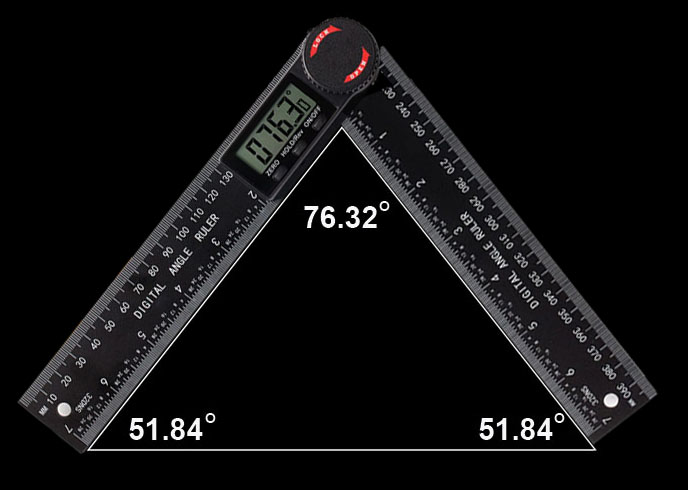# For a quick rough visual angle check

Set the digital angle ruler to 76.3° and turn the knob to lock that angle in place.

Place the ruler over the crystal's termination angle for a rough visual check

Repeat the same procedure for checking the other termination end for the 60.0°.

# Examples: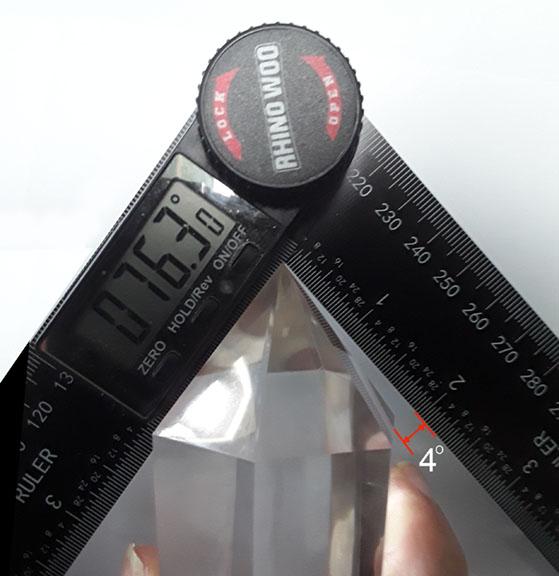76.3° rough quick check on 6 sided looks very off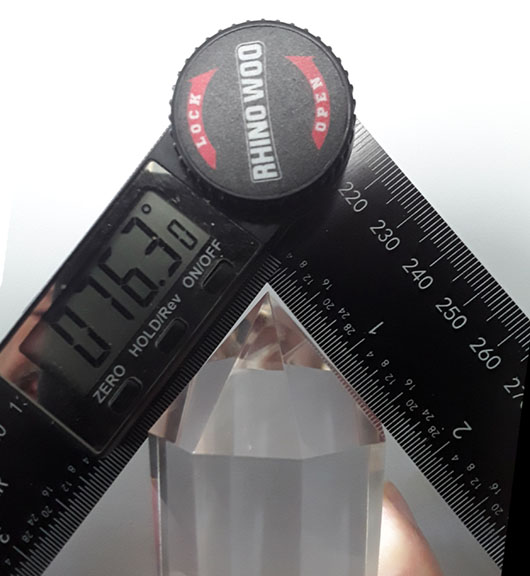76.3° rough quick check on 8 sided looks almost close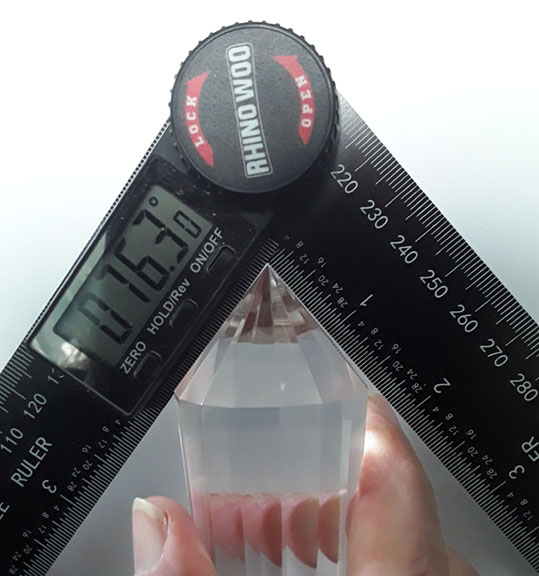76.3° rough quick check on 16 sided looks very good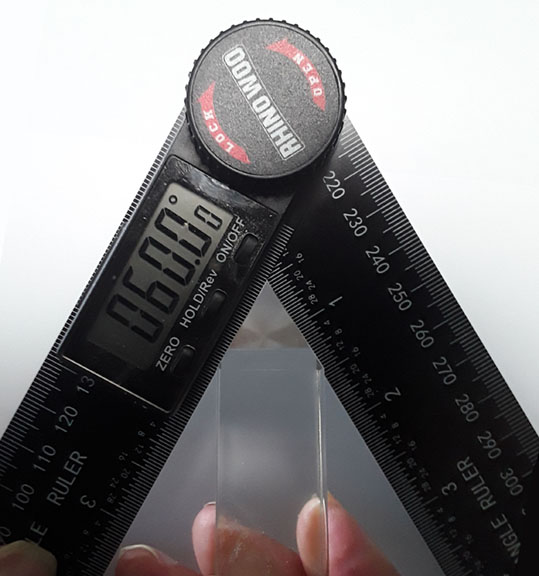60.0° Rough quick check on 4 sided looks perfect

Note: Dr Marcel Vogel personally cut this 4 sided crystal above for me as a gift

# Measurement of the Angle:

In order to find out the pyramidal angle, you need to put both rulers completely flush against the flat opposing pyramidal sides and read the angle measured in order to calculate the degrees off. Note this works only for all even number sided crystals so that you have two opposing pyramidal surfaces to measure (i.e. 4,6,8,12,16,24 sided etc.)

## Instructions:

Note as you push both rulers down flush against the pyramidal sides, make sure the apex point of the crystal is wedged in between the rulers with the absolute minimum of light appearing above the crystals apex with both rulers pushed down flush on both sides. Use a sheet of white paper behind to help see and make sure that both sides are flush with the rulers and no light is appearing from the sides as well.

For measuring the 51.84° transponding receiver end the correct reading is 76.3°

# Examples: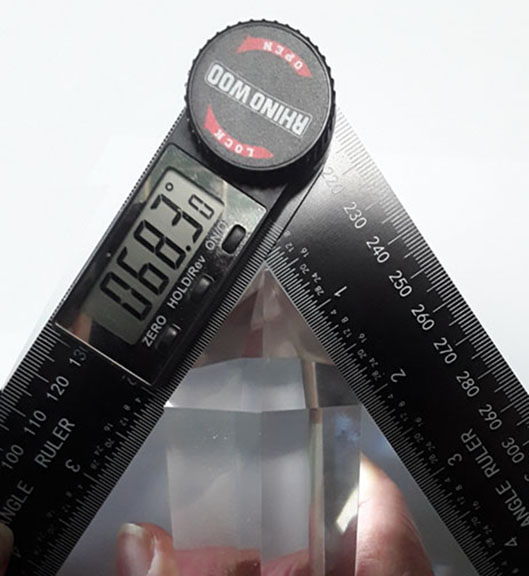You measure 68.3°

You subtract 68.3 from 76.32 = 8.02

In order to find the degrees off on one of the two sides you divide by 2

8.02 /2 = 4.01 degrees too steep of an angle difference from ideal

Add the 4.01 to 51.84 will give you the angle of the slope on one side

4.01 + 51.84 = The angle is 55.85 degrees being too steep at 4.01 degrees off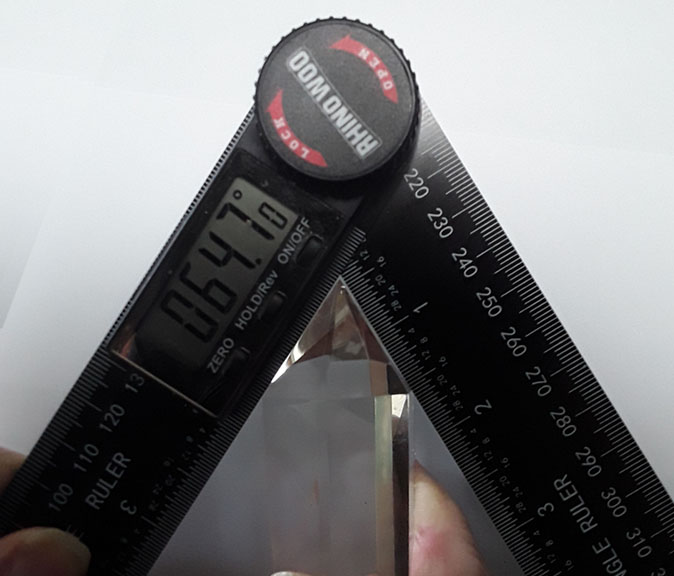You measure 64.7°

You subtract 64.7 from 76.32 = 11.62

In order to find the degrees off on one of the two sides you divide by 2

11.62 /2 = 5.81 degrees too steep of an angle difference from ideal

Add the 5.81 to 51.84 will give you the angle of the slope on one side

5.81 + 51.84 = The angle is 57.65 degrees being too steep at 5.81 degrees off

An example of a negative reading meaning the pyramidal angle is not steep enough

You measure 79.5°

You subtract 79.5 from 76.32 = -3.18 (a negative number)

In order to find the degrees off on one of the two sides you divide by 2

-3.18 /2 = -1.59 degrees not steep enough of an angle difference from ideal

Add the -1.59 to 51.84 will give you the angle of the slope on one side

-1.59 + 51.84 = The angle is 50.25 degrees being not steep enough at -1.59 degrees off

The same exact procedure applies to the other termination end

For measuring the 60.00° emitter sending end the correct reading is set to 60.0° instead of 76.3° for the calculations to determine the termination angle.

MarcelVogel.org